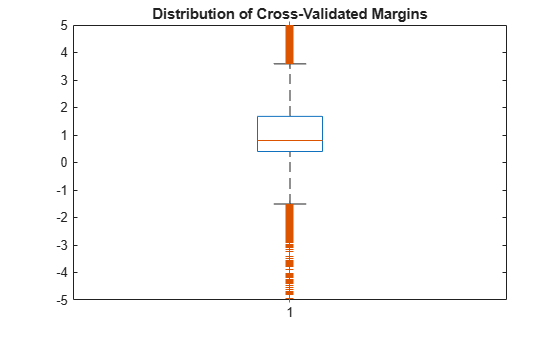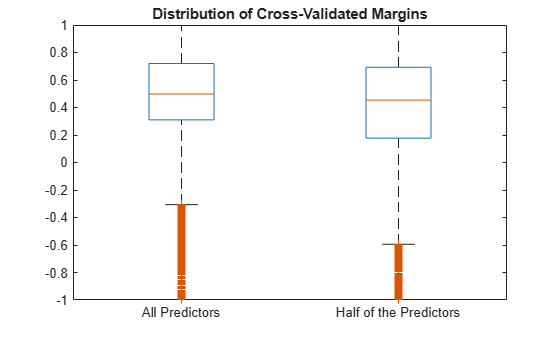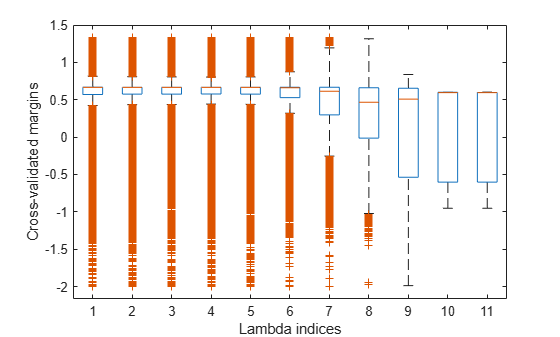# kfoldMargin

Classification margins for observations not used in training

## Syntax

``m = kfoldMargin(CVMdl)``
``m = kfoldMargin(CVMdl,Name,Value)``

## Description

example

````m = kfoldMargin(CVMdl)` returns the cross-validated classification margins obtained by `CVMdl`, which is a cross-validated, error-correcting output codes (ECOC) model composed of linear classification models. That is, for every fold, `kfoldMargin` estimates the classification margins for observations that it holds out when it trains using all other observations.`m` contains classification margins for each regularization strength in the linear classification models that comprise `CVMdl`.```

example

````m = kfoldMargin(CVMdl,Name,Value)` uses additional options specified by one or more `Name,Value` pair arguments. For example, specify a decoding scheme or verbosity level.```

## Input Arguments

expand all

Cross-validated, ECOC model composed of linear classification models, specified as a `ClassificationPartitionedLinearECOC` model object. You can create a `ClassificationPartitionedLinearECOC` model using `fitcecoc` and by:

1. Specifying any one of the cross-validation, name-value pair arguments, for example, `CrossVal`

2. Setting the name-value pair argument `Learners` to `'linear'` or a linear classification model template returned by `templateLinear`

To obtain estimates, kfoldMargin applies the same data used to cross-validate the ECOC model (`X` and `Y`).

### Name-Value Arguments

Specify optional pairs of arguments as `Name1=Value1,...,NameN=ValueN`, where `Name` is the argument name and `Value` is the corresponding value. Name-value arguments must appear after other arguments, but the order of the pairs does not matter.

Before R2021a, use commas to separate each name and value, and enclose `Name` in quotes.

Binary learner loss function, specified as the comma-separated pair consisting of `'BinaryLoss'` and a built-in loss function name or function handle.

• This table contains names and descriptions of the built-in functions, where yj is the class label for a particular binary learner (in the set {-1,1,0}), sj is the score for observation j, and g(yj,sj) is the binary loss formula.

ValueDescriptionScore Domaing(yj,sj)
`'binodeviance'`Binomial deviance(–∞,∞)log[1 + exp(–2yjsj)]/[2log(2)]
`'exponential'`Exponential(–∞,∞)exp(–yjsj)/2
`'hamming'`Hamming[0,1] or (–∞,∞)[1 – sign(yjsj)]/2
`'hinge'`Hinge(–∞,∞)max(0,1 – yjsj)/2
`'linear'`Linear(–∞,∞)(1 – yjsj)/2
`'logit'`Logistic(–∞,∞)log[1 + exp(–yjsj)]/[2log(2)]
`'quadratic'`Quadratic[0,1][1 – yj(2sj – 1)]2/2

The software normalizes the binary losses such that the loss is 0.5 when yj = 0. Also, the software calculates the mean binary loss for each class.

• For a custom binary loss function, e.g., `customFunction`, specify its function handle `'BinaryLoss',@customFunction`.

`customFunction` should have this form

`bLoss = customFunction(M,s)`
where:

• `M` is the K-by-B coding matrix stored in `Mdl.CodingMatrix`.

• `s` is the 1-by-B row vector of classification scores.

• `bLoss` is the classification loss. This scalar aggregates the binary losses for every learner in a particular class. For example, you can use the mean binary loss to aggregate the loss over the learners for each class.

• K is the number of classes.

• B is the number of binary learners.

For an example of passing a custom binary loss function, see Predict Test-Sample Labels of ECOC Model Using Custom Binary Loss Function.

By default, if all binary learners are linear classification models using:

• SVM, then `BinaryLoss` is `'hinge'`

• Logistic regression, then `BinaryLoss` is `'quadratic'`

Example: `'BinaryLoss','binodeviance'`

Data Types: `char` | `string` | `function_handle`

Decoding scheme that aggregates the binary losses, specified as the comma-separated pair consisting of `'Decoding'` and `'lossweighted'` or `'lossbased'`. For more information, see Binary Loss.

Example: `'Decoding','lossbased'`

Estimation options, specified as the comma-separated pair consisting of `'Options'` and a structure array returned by `statset`.

To invoke parallel computing:

• You need a Parallel Computing Toolbox™ license.

• Specify `'Options',statset('UseParallel',true)`.

Verbosity level, specified as the comma-separated pair consisting of `'Verbose'` and `0` or `1`. `Verbose` controls the number of diagnostic messages that the software displays in the Command Window.

If `Verbose` is `0`, then the software does not display diagnostic messages. Otherwise, the software displays diagnostic messages.

Example: `'Verbose',1`

Data Types: `single` | `double`

## Output Arguments

expand all

Cross-validated classification margins, returned as a numeric vector or matrix.

`m` is n-by-L, where n is the number of observations in `X` and L is the number of regularization strengths in `Mdl` (that is, `numel(Mdl.Lambda)`).

`m(i,j)` is the cross-validated classification margin of observation i using the ECOC model, composed of linear classification models, that has regularization strength `Mdl.Lambda(j)`.

## Examples

expand all

`load nlpdata`

`X` is a sparse matrix of predictor data, and `Y` is a categorical vector of class labels.

For simplicity, use the label 'others' for all observations in `Y` that are not `'simulink'`, `'dsp'`, or `'comm'`.

`Y(~(ismember(Y,{'simulink','dsp','comm'}))) = 'others';`

Cross-validate a multiclass, linear classification model.

```rng(1); % For reproducibility CVMdl = fitcecoc(X,Y,'Learner','linear','CrossVal','on');```

`CVMdl` is a `ClassificationPartitionedLinearECOC` model. By default, the software implements 10-fold cross validation. You can alter the number of folds using the `'KFold'` name-value pair argument.

Estimate the k-fold margins.

```m = kfoldMargin(CVMdl); size(m)```
```ans = 1×2 31572 1 ```

`m` is a 31572-by-1 vector. `m(j)` is the average of the out-of-fold margins for observation `j`.

Plot the k-fold margins using box plots.

```figure; boxplot(m); h = gca; h.YLim = [-5 5]; title('Distribution of Cross-Validated Margins')```One way to perform feature selection is to compare k-fold margins from multiple models. Based solely on this criterion, the classifier with the larger margins is the better classifier.

Load the NLP data set. Preprocess the data as in Estimate k-Fold Cross-Validation Margins, and orient the predictor data so that observations correspond to columns.

```load nlpdata Y(~(ismember(Y,{'simulink','dsp','comm'}))) = 'others'; X = X';```

Create these two data sets:

• `fullX` contains all predictors.

• `partX` contains 1/2 of the predictors chosen at random.

```rng(1); % For reproducibility p = size(X,1); % Number of predictors halfPredIdx = randsample(p,ceil(0.5*p)); fullX = X; partX = X(halfPredIdx,:);```

Create a linear classification model template that specifies optimizing the objective function using SpaRSA.

`t = templateLinear('Solver','sparsa');`

Cross-validate two ECOC models composed of binary, linear classification models: one that uses the all of the predictors and one that uses half of the predictors. Indicate that observations correspond to columns.

```CVMdl = fitcecoc(fullX,Y,'Learners',t,'CrossVal','on',... 'ObservationsIn','columns'); PCVMdl = fitcecoc(partX,Y,'Learners',t,'CrossVal','on',... 'ObservationsIn','columns');```

`CVMdl` and `PCVMdl` are `ClassificationPartitionedLinearECOC` models.

Estimate the k-fold margins for each classifier. Plot the distribution of the k-fold margins sets using box plots.

```fullMargins = kfoldMargin(CVMdl); partMargins = kfoldMargin(PCVMdl); figure; boxplot([fullMargins partMargins],'Labels',... {'All Predictors','Half of the Predictors'}); h = gca; h.YLim = [-1 1]; title('Distribution of Cross-Validated Margins')```The distributions of the k-fold margins of the two classifiers are similar.

To determine a good lasso-penalty strength for a linear classification model that uses a logistic regression learner, compare distributions of k-fold margins.

Load the NLP data set. Preprocess the data as in Feature Selection Using k-fold Margins.

```load nlpdata Y(~(ismember(Y,{'simulink','dsp','comm'}))) = 'others'; X = X';```

Create a set of 11 logarithmically-spaced regularization strengths from $1{0}^{-8}$ through $1{0}^{1}$.

`Lambda = logspace(-8,1,11);`

Create a linear classification model template that specifies using logistic regression with a lasso penalty, using each of the regularization strengths, optimizing the objective function using SpaRSA, and reducing the tolerance on the gradient of the objective function to `1e-8`.

```t = templateLinear('Learner','logistic','Solver','sparsa',... 'Regularization','lasso','Lambda',Lambda,'GradientTolerance',1e-8);```

Cross-validate an ECOC model composed of binary, linear classification models using 5-fold cross-validation and that

```rng(10); % For reproducibility CVMdl = fitcecoc(X,Y,'Learners',t,'ObservationsIn','columns','KFold',5)```
```CVMdl = ClassificationPartitionedLinearECOC CrossValidatedModel: 'LinearECOC' ResponseName: 'Y' NumObservations: 31572 KFold: 5 Partition: [1x1 cvpartition] ClassNames: [comm dsp simulink others] ScoreTransform: 'none' Properties, Methods ```

`CVMdl` is a `ClassificationPartitionedLinearECOC` model.

Estimate the k-fold margins for each regularization strength. The scores for logistic regression are in [0,1]. Apply the quadratic binary loss.

```m = kfoldMargin(CVMdl,'BinaryLoss','quadratic'); size(m)```
```ans = 1×2 31572 11 ```

`m` is a 31572-by-11 matrix of cross-validated margins for each observation. The columns correspond to the regularization strengths.

Plot the k-fold margins for each regularization strength.

```figure; boxplot(m) ylabel('Cross-validated margins') xlabel('Lambda indices')```Several values of `Lambda` yield similarly high margin distribution centers with low spreads. Higher values of `Lambda` lead to predictor variable sparsity, which is a good quality of a classifier.

Choose the regularization strength that occurs just before the margin distribution center starts decreasing and spread starts increasing.

`LambdaFinal = Lambda(5);`

Train an ECOC model composed of linear classification model using the entire data set and specify the regularization strength `LambdaFinal`.

```t = templateLinear('Learner','logistic','Solver','sparsa',... 'Regularization','lasso','Lambda',Lambda(5),'GradientTolerance',1e-8); MdlFinal = fitcecoc(X,Y,'Learners',t,'ObservationsIn','columns');```

To estimate labels for new observations, pass `MdlFinal` and the new data to `predict`.